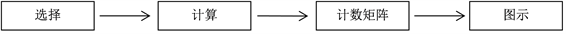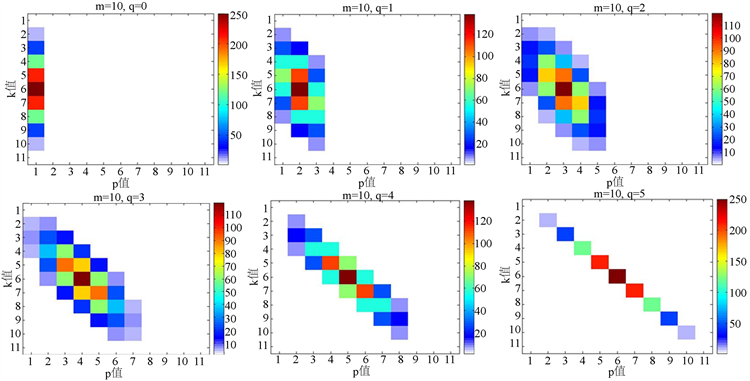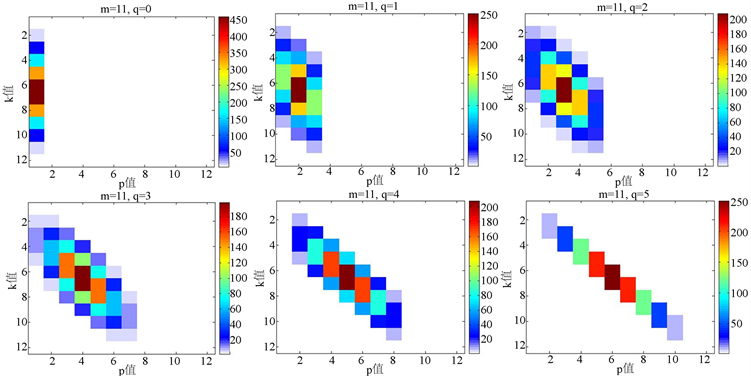﻿ 基于变值测量对三项式组合系数的多维可视化探索

# 基于变值测量对三项式组合系数的多维可视化探索 Multidimensional Visualization Exploration of Trinomial Combination Coef-ficient Based on Variant Measurement

Abstract: The binomial and trinomial combination counting calculation mode plays a core role in data description and data analysis of probability statistics, artificial intelligence, and big data. In this paper, the variable measurement is used to calculate and project the multi-dimensional distribution of the trinomial combination counting coefficient. Based on the binomial combination equation and the quaternary variable metric, the binomial combination coefficients are decomposed and transformed to form a trinomial combination coefficient equation, and the two-dimensional quantization counting matrix is obtained by the combination number calculation method. The relevant numerical calculation results are converted into statistical two-dimensional histograms and displayed in a two-dimensional color map. In this paper, the results of spatial symmetry and distribution diversity of the trinomial coefficients are exhibited by using multiple graphical results. From the variation and invariant characteristics of the trinomial combination coefficient distribution, the clustering distribution characteristics of various combined transformations are systematically explored.

1. 引言

2. 基本理论

2.1. 二项式系数

$\left(\begin{array}{c}n\\ i\end{array}\right)=\frac{n!}{i!\left(n-i\right)!}$ (1)

2.2. 范德蒙德综合式

(范德蒙德综合式)对所有整数n，有

${\sum }_{k}\left(\begin{array}{c}r\\ k\end{array}\right)\left(\begin{array}{c}s\\ n-k\end{array}\right)=\left(\begin{array}{c}r+s\\ n\end{array}\right)$ (2)

2.3. 基本测量公式

$\left(\begin{array}{c}m\\ p\end{array}\right)={\sum }_{p}\left(\begin{array}{c}m-2q\\ p-k\end{array}\right)\left(\begin{array}{c}2q\\ k\end{array}\right)$ (3)

3. 计算模型Figure 1. Curve: Trinomial coefficient solution processing model

4. 结果展示

4.1. 计数矩阵

· 当m = 10，m = 11，q = 0时有：Table 1. Resulting data of trinomial coefficients

*注：表中只显示有数据的列，未显示的数据列，其列值均为0。

· 当m = 10，m = 11，q = 2时有：Table 2. Resulting data of trinomial coefficients

*注：表中只显示有数据的列，未显示的数据列，其列值均为0。

· 当m = 10，m = 11，q = 4时有：Table 3. Resulting data of trinomial coefficients

*注：表中只显示有数据的列，未显示的数据列，其列值均为0。

4.2. 图示

4.2.1. m = 10, q值变换Figure 2. Curve: q value conversion result icon

4.2.2. m = 11, q值变换Figure 3. Curve: q value conversion result icon

5. 结果分析

6. 总结

 宋桢桢, 及万会. 由裂项法导出二项式系数级数[J]. 西南民族大学学报(自然科学版), 2013, 39(5): 709-717.

 毛经中. 组合数学基础[M]. 武汉: 华中师范大学出版社, 1990.

 Hall, M. (1986) Combinatorial Theory. 2nd Edition, Wiley Interscience, New York.

 Chen, J.R. (2012) Combinatorial Mathematics. Harbin Institute of Technology Press, Harbin.

 Gould, H.W. (1972) Combinatorial Identities. Morgantown Printing and Binding Company, Morganton.

 Stanton, D. and White, D. (1986) Constructive Combinatorics. Springer, New York.
https://doi.org/10.1007/978-1-4612-4968-9

 李秦. 基于Matlab的数学可视化问题研究及实践[J]. 兰州交通大学学报, 2013, 32(4): 27-30.

 郑智捷, 郑昊航. 变值测量结构及其可视化统计分布[J]. 光子学报, 2011, 40(9): 1397-1404.

 Zheng, J. (2011) Conditional Probability Statistical Distributions in Variant Measurement Simulations. Acta Photonica Sinica, 40, 1662-1666.
https://doi.org/10.3788/gzxb20114011.1662

 郑智捷. 多元逻辑函数的基础等价变值表示[J]. 云南民族大学学报(自然科学版), 2011, 20(5): 396-397.

 Knuth, D.E. (1998) Art of Computer Programming, the: Volume 1: Fundamental Algorithms, 3rd Edition. China Machine Press, Beijing.

 Gould, H.W. (1956) Some Generalizations of Vandermonde’s Convolution. American Mathematical Monthly, 63, 84-91.
https://doi.org/10.1080/00029890.1956.11988763

 Zheng, J. (2019) Variant Logic Construction under Permutation and Complementary Operations on Binary Logic. In: Zheng, J., Ed., Variant Construction from Theoretical Foundation to Applications, Springer, Singapore, 3-21.
https://doi.org/10.1007/978-981-13-2282-2_1

Top